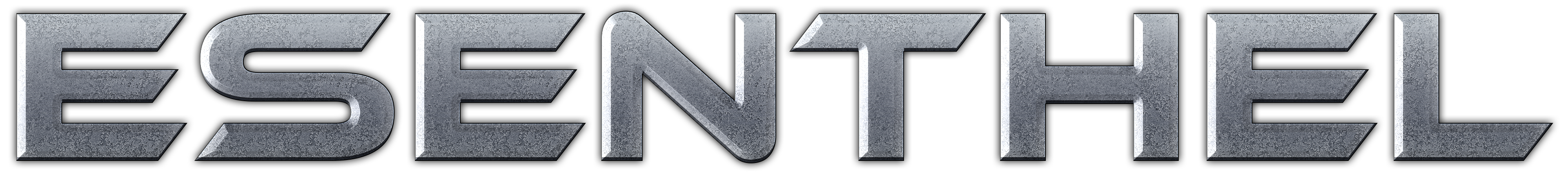About Store Forum Documentation ContactHow to make grid on the terrain just like in World Editor Author Message
XeriosMember

 Post: #1 How to make grid on the terrain just like in World Editor I was wondering how I'm supposed to add a secondary grid texture to an existing terrain ( loaded from .world file ) I'm trying to place a grid on the terrain something similar to the one in the World Editor when you're in "path editing mode" Thanks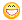08-22-2009 01:44 AM
Esenthel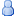Post: #2 Re: How to make grid on the terrain just like in World Editor I'll add this kind of functionality soon (because it will require heightmap access for faster rendering) but you can use Physics.ray to get the heightmap position and draw quads using VI (vertex index buffer) after calling "Renderer(Render);"
08-22-2009 11:41 AM
RodrigoMember

 Post: #3 Re: How to make grid on the terrain just like in World Editor I have an similar problem, but i want an hexagonal grid. how to do that?
09-02-2009 08:35 PM
AndrewBGSMemberPost: #4 RE: How to make grid on the terrain just like in World Editor I still didn't get the answer to the simple square grid problem; Can't I somehow display the grid without adding an extra texture? (heightmap isn't a problem for me, I want this in a perfectly flat world)
09-04-2013 08:05 AM
FexBronze SupporterPost: #5 RE: How to make grid on the terrain just like in World Editor Here is how I draw a grid over the terrain, it is not very efficient performance wise. Call DrawGrid() in RM_BLEND mode. If you want to make it look more like the world editor try playing with color (use transparency) and width of Edge.draw. Code: ```void DrawGrid() {   D.alpha(ALPHA_BLEND_DEC);   Int draw_distance = 30;   Flt height_above_terrain = 0.1; // meters the grid is placed above the terrain, this helps with clipping on steep areas      RectI  ah = RectI(VecI2(-draw_distance, -draw_distance), VecI2(draw_distance, draw_distance)); // area heightmap   for(int hy=ah.min.y; hy<=ah.max.y; hy++) // iterate area heightmap   for(int hx=ah.min.x; hx<=ah.max.x; hx++)   {       Vec pos =Vec(Floor(Plr.pos()));       pos = Vec(pos.x, 0, pos.z); // remove the hieght value, we will set that from the heightmap, not from the player              Quad Q;       Q.set(pos + Vec(hx, Game.World.hmHeight(pos.xz()+Vec2(hx, hy))+height_above_terrain, hy), pos + Vec(hx+1, Game.World.hmHeight(pos.xz()+Vec2(hx+1, hy))+height_above_terrain, hy), pos + Vec(hx, Game.World.hmHeight(pos.xz()+Vec2(hx, hy+1))+height_above_terrain, hy+1), pos + Vec(hx+1, Game.World.hmHeight(pos.xz()+Vec2(hx+1, hy+1))+height_above_terrain, hy+1));              if(Dist(Plr.pos(), Q.center()) < 10)       {          Edge(Q.p,Q.p).draw(GREEN);          Edge(Q.p,Q.p).draw(GREEN);          Edge(Q.p,Q.p).draw(GREEN);          Edge(Q.p,Q.p).draw(GREEN);       }       else       {          Edge(Q.p,Q.p).draw(RED);          Edge(Q.p,Q.p).draw(RED);          Edge(Q.p,Q.p).draw(RED);          Edge(Q.p,Q.p).draw(RED);       }      } }```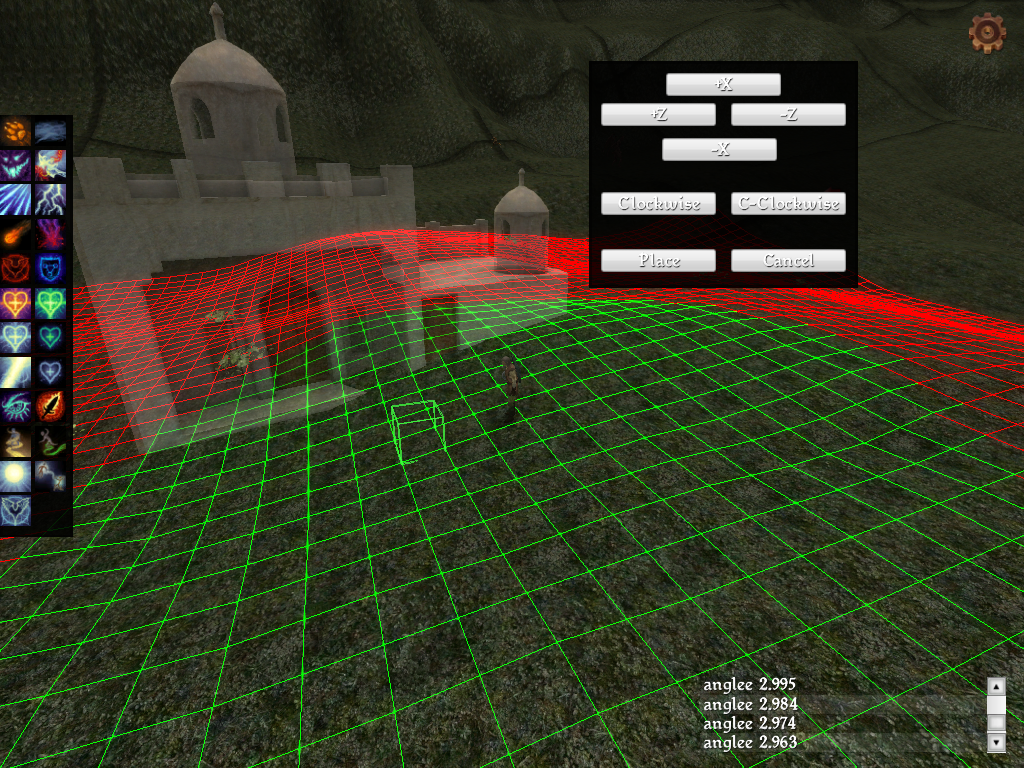12-21-2013 05:27 PM
candamMember

 Post: #6 RE: How to make grid on the terrain just like in World Editor (12-21-2013 05:27 PM)Fex Wrote:  Here is how I draw a grid over the terrain, it is not very efficient performance wise. Call DrawGrid() in RM_BLEND mode. If you want to make it look more like the world editor try playing with color (use transparency) and width of Edge.draw. Code: ```void DrawGrid() {   D.alpha(ALPHA_BLEND_DEC);   Int draw_distance = 30;   Flt height_above_terrain = 0.1; // meters the grid is placed above the terrain, this helps with clipping on steep areas      RectI  ah = RectI(VecI2(-draw_distance, -draw_distance), VecI2(draw_distance, draw_distance)); // area heightmap   for(int hy=ah.min.y; hy<=ah.max.y; hy++) // iterate area heightmap   for(int hx=ah.min.x; hx<=ah.max.x; hx++)   {       Vec pos =Vec(Floor(Plr.pos()));       pos = Vec(pos.x, 0, pos.z); // remove the hieght value, we will set that from the heightmap, not from the player              Quad Q;       Q.set(pos + Vec(hx, Game.World.hmHeight(pos.xz()+Vec2(hx, hy))+height_above_terrain, hy), pos + Vec(hx+1, Game.World.hmHeight(pos.xz()+Vec2(hx+1, hy))+height_above_terrain, hy), pos + Vec(hx, Game.World.hmHeight(pos.xz()+Vec2(hx, hy+1))+height_above_terrain, hy+1), pos + Vec(hx+1, Game.World.hmHeight(pos.xz()+Vec2(hx+1, hy+1))+height_above_terrain, hy+1));              if(Dist(Plr.pos(), Q.center()) < 10)       {          Edge(Q.p,Q.p).draw(GREEN);          Edge(Q.p,Q.p).draw(GREEN);          Edge(Q.p,Q.p).draw(GREEN);          Edge(Q.p,Q.p).draw(GREEN);       }       else       {          Edge(Q.p,Q.p).draw(RED);          Edge(Q.p,Q.p).draw(RED);          Edge(Q.p,Q.p).draw(RED);          Edge(Q.p,Q.p).draw(RED);       }      } }```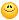This topic was years ago but I like the sense of helping others Thank you for helping people bro12-21-2013 08:51 PM
 « Next Oldest | Next Newest »